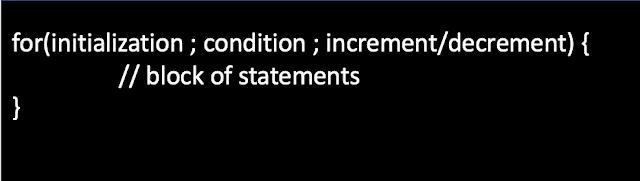# Increment for Loop by 2 in Java with examples

In this blog post, I will be sharing how to increment for loop by 2 in Java with examples. Let's dive deep into the topic:

Q. Can a for loop increment or decrement by more than one in Java?

The answer to the above question is yes. Using the assignment operator += we can increment or decrement by more than one as shown below in the examples.

Note: Use i+=2 in case we need to increment for loop by 2 whereas use i-=2 in case we need to decrement for loop by 2 in Java.

## for loop syntax

The for loop consists of 3 parts:

1. Initialization
2. Condition
3. Increment or Decrement

The syntax of the for loop is shown below in the image:1. Initialization: The initialization statement executes only one time i.e. at the start of the loop.

2. Condition: The condition of the for loop gets evaluated in each iteration and must return a boolean value. For loop will continue to execute the block of statements in the loop unless the condition returns false.

3. Increment or Decrement: This statement gets executed each time the condition of the for loop is true.

## Increment for Loop by 2 in Java with examples

To increment for loop by 2 in Java, we only need to change the increment/decrement part of the for loop.

### Examples

#### Increment for loop by 2 example

In the below example, we are printing odd numbers from 1 to 15.

```public class PrintOddNumbers {
public static void main(String args[]) {
for(int i=1; i <= 15 ; i+=2)
{
System.out.print(i + " ");
}
}
}
```

Output:
1 3 5 7 9 11 13 15

#### Decrement for loop by 2 example

In the below example, we are printing odd numbers in reverse order i.e. from 15 to 1.

```public class PrintOddNumbers2 {
public static void main(String args[]) {
for(int i=15; i >= 1 ; i-=2)
{
System.out.print(i + " ");
}
}
}
```

Output:
15 13 11 9 7 5 3 1

## Increment for Loop by n in Java with examples

Just like above we can increment for loop by n i.e. 2,3,4,5,6. We just need to replace the increment or decrement part of the for loop by i+=n as shown below in the example.

Simple Java program where we will increment for loop by 5.

```public class IncrementForLoopBy5 {
public static void main(String args[]) {
for(int i=1; i < 27 ; i+=5)
{
System.out.print(i + " ");
}
}
}
```

Output:
1 6 11 16 21 26

That's all for today. Please mention in the comments if you have any questions related to how to increment for loop by 2 in Java with examples.